# Angles and Arcs Recognize major arcs minor arcs

• Slides: 14Angles and Arcs • Recognize major arcs, minor arcs, semicircles, and central angles and their measures. • Find arc length. An artist’s rendering of Larry Niven’s Ringworld.Two artist’s conceptions of views from the surface of Ringworld.ANGLES AND ARCS A central angle has the center of a circle as its vertex, and its sides contain two radii of the circle. The sum of the measures of the angles around the center of a circle is 360°.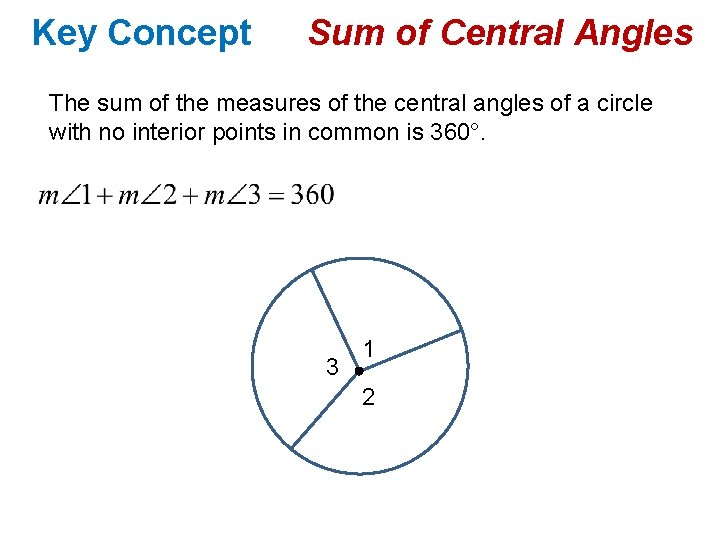Key Concept Sum of Central Angles The sum of the measures of the central angles of a circle with no interior points in common is 360°. 3 1 2Example 1 Measure of Central Angles a) Find m AOB B 25 x° A O E b) Find m AOE 3 x° C 2 x° DKey Concept Arcs of a Circle Minor Arc Major Arc A D E B Semicircle 110° C J 60° G N F Usually named using the letters of the two endpoints. AC K Named by the letters of the two endpoints and another point on the arc. DFE M L Named by the letters of the two endpoints and another point on the arc. JML and JKL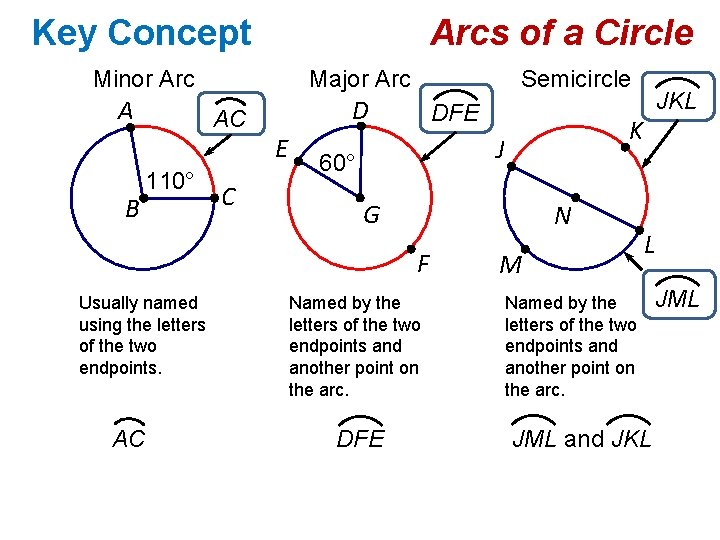Key Concept Arcs of a Circle Minor Arc A B Major Arc AC 110° C Semicircle D E DFE G N F Usually named using the letters of the two endpoints. AC K J 60° Named by the letters of the two endpoints and another point on the arc. DFE JKL M L Named by the letters of the two endpoints and another point on the arc. JML and JKL JML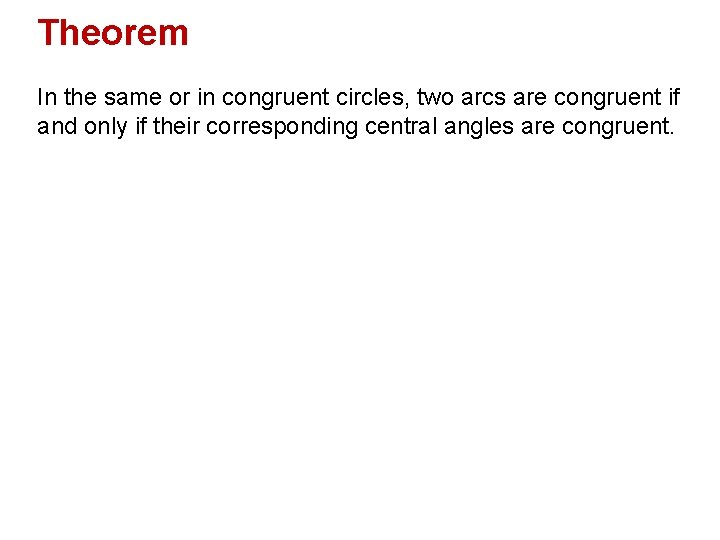Theorem In the same or in congruent circles, two arcs are congruent if and only if their corresponding central angles are congruent.Postulate Arc Addition Postulate The measure of an arc formed by two adjacent arcs is the sum of the measures of the two arcs. P S Q R In circle S, m. PQ + m. QR = m. PQR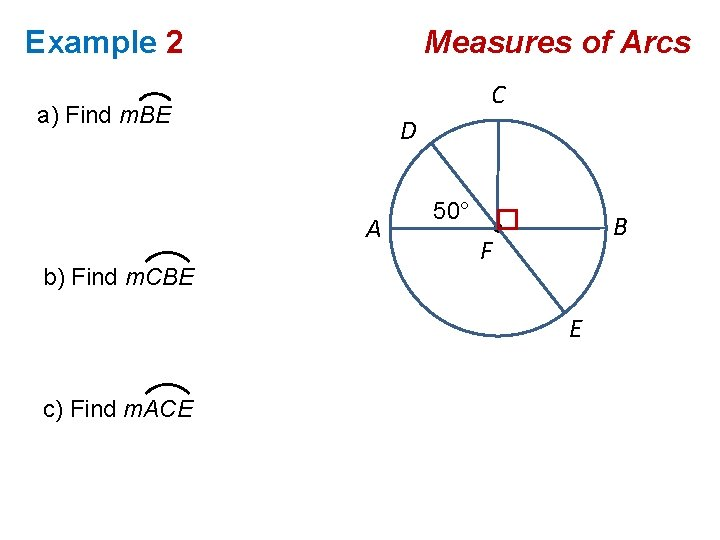Example 2 Measures of Arcs C a) Find m. BE D A b) Find m. CBE 50° B F E c) Find m. ACE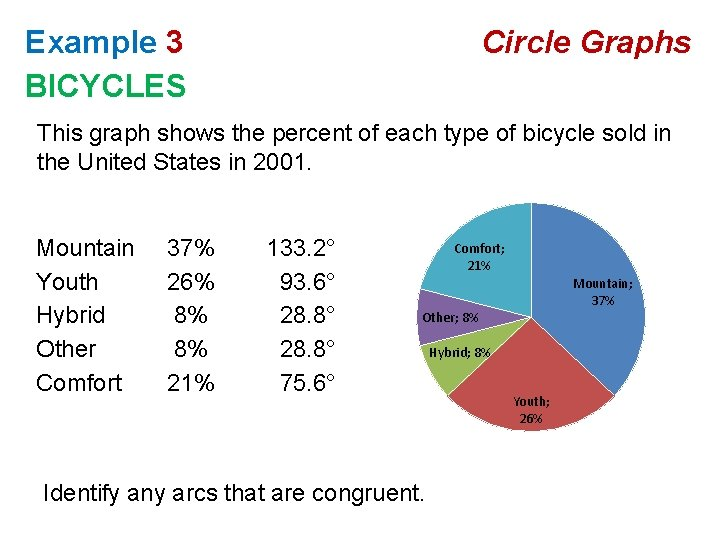Example 3 BICYCLES Circle Graphs This graph shows the percent of each type of bicycle sold in the United States in 2001. Mountain Youth Hybrid Other Comfort 37% 26% 8% 8% 21% 133. 2° 93. 6° 28. 8° 75. 6° Comfort; 21% Mountain; 37% Other; 8% Identify any arcs that are congruent. Hybrid; 8% Youth; 26%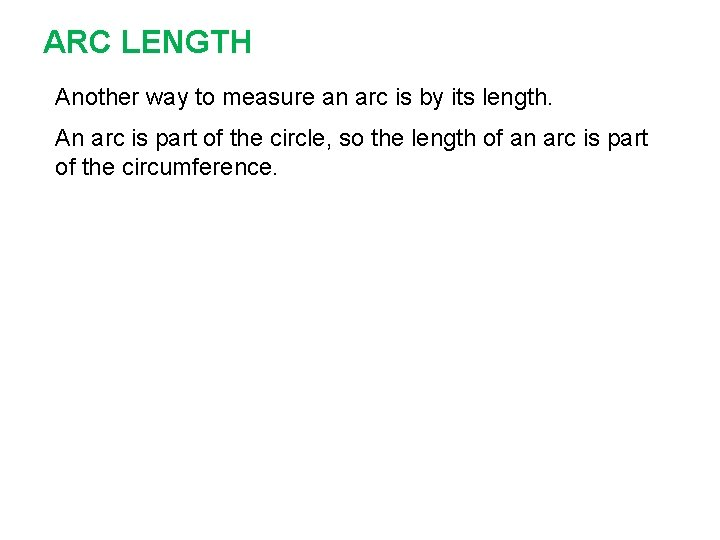ARC LENGTH Another way to measure an arc is by its length. An arc is part of the circle, so the length of an arc is part of the circumference.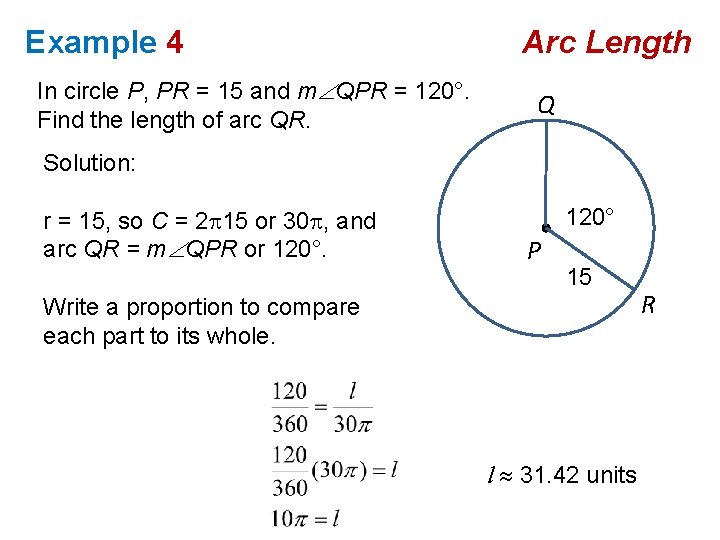Example 4 In circle P, PR = 15 and m QPR = 120°. Find the length of arc QR. Arc Length Q Solution: r = 15, so C = 2 15 or 30 , and arc QR = m QPR or 120° P 15 Write a proportion to compare each part to its whole. l 31. 42 units R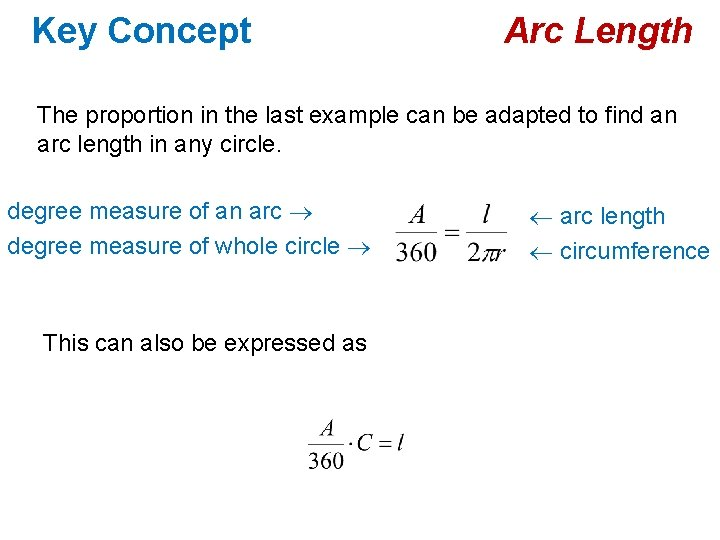Key Concept Arc Length The proportion in the last example can be adapted to find an arc length in any circle. degree measure of an arc degree measure of whole circle This can also be expressed as ¬ arc length ¬ circumference